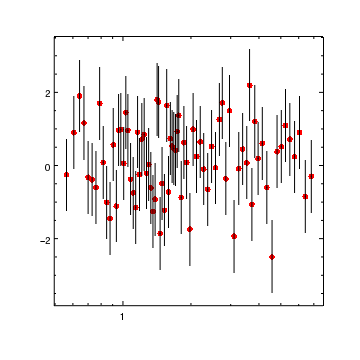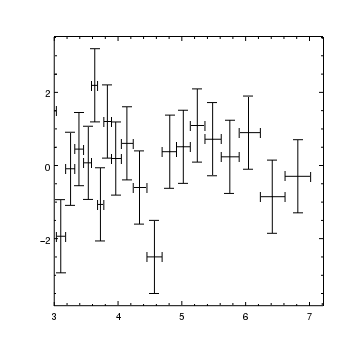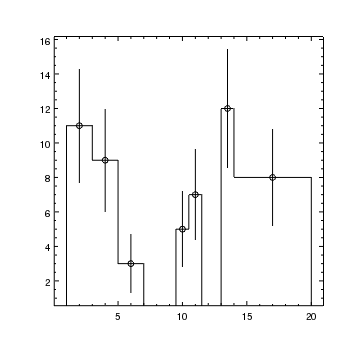# Gallery: Error bars

## 1) Symmetric Y errors

Curves and histograms can display error bars; if given a single value, as in this example, then symmetric Y errors are assumed.Version: Postscript; PDF
```crv = ChipsCurve()
crv.line.style = "noline"
set_curve(["symbol.style","circle","symbol.size",3,"symbol.color","red"])
log_scale(X_AXIS)
```

The error bars can be drawn in a different color to the curve: the available properties of error bars for curves can be seen by saying:

```chips> get_curve().err

caplength = 10
color = default
down = True
left = False
right = False
style = line
thickness = 1.0
up = True

```

## 2) Errors for both the X and Y axes

Here we display error bars for both the X and Y axes and show how to change the error style so that the errors are drawn with a bar or cap at the end.Version: Postscript; PDF
```tbl = read_file("spectrum.fits[residuals]")
x = copy_colvals(tbl,"x")
y = copy_colvals(tbl,"y")
dylo = copy_colvals(tbl,"dylo")
dyhi = copy_colvals(tbl,"dyhi")
dxlo = copy_colvals(tbl,"dxlo")
dxhi = copy_colvals(tbl,"dxhi")
crv = ChipsCurve()
crv.line.style = "noline"
crv.symbol.style = "none"
crv.err.style = "capped"
set_curve(crv)
limits(X_AXIS,3,AUTO)
```

In order to display X errors, then four error arrays must be given; one each for the lower and upper errors for the Y and then X axes, even if the errors are symmetrical. The only way this data can be specified is by reading in the data into arrays and then using them in the add_curve call; the following command will not create the same plot:

```add_curve("spectrum.fits[residuals][cols x,y,dylo,dyhi,dxlo,dxhi]")
```

## 3) A histogram with errors and symbols

Histograms can also display error bars, but only for the Y axis.Version: Postscript; PDF
```tbl = read_file("histogram.fits")
xlo = copy_colvals(tbl,"xlo")
xhi = copy_colvals(tbl,"xhi")
y = copy_colvals(tbl,"y")
dylo = copy_colvals(tbl,"dylo")
dyhi = copy_colvals(tbl,"dyhi")